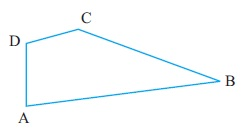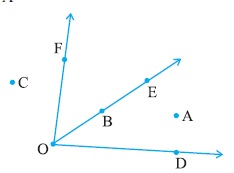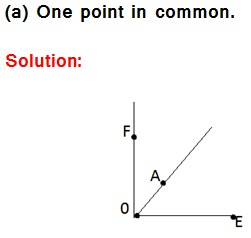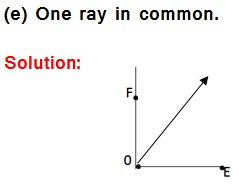Study Materials

# NCERT Solutions for Class 6th Mathematics

Page 3 of 6

## Chapter 4. Basic Geometrical Ideas

### Exercise 4.3

Exercise 4.3

Q1. Name the angles in the given figure.Q2. In the given diagram, name the point(s)
(a) In the interior of ∠DOE
(b) In the exterior of ∠EOF
(c) On ∠EOF(a) In the interior of DOE

Solution: Point A

(b) In the exterior of EOF.

Solution: Point C

(c) On EOF

Solution: Point B

Q3. Draw rough diagrams of two angles such that they have
(a) One point in common.
(b) Two points in common.
(c) Three points in common.
(d) Four points in common.
(e) One ray in common.

Solution:Page 3 of 6

Chapter Contents: